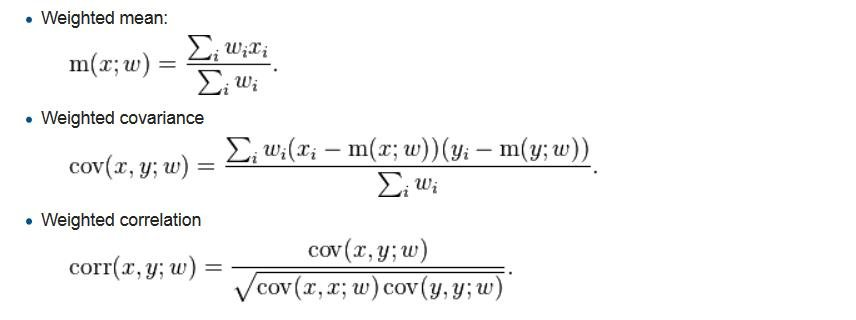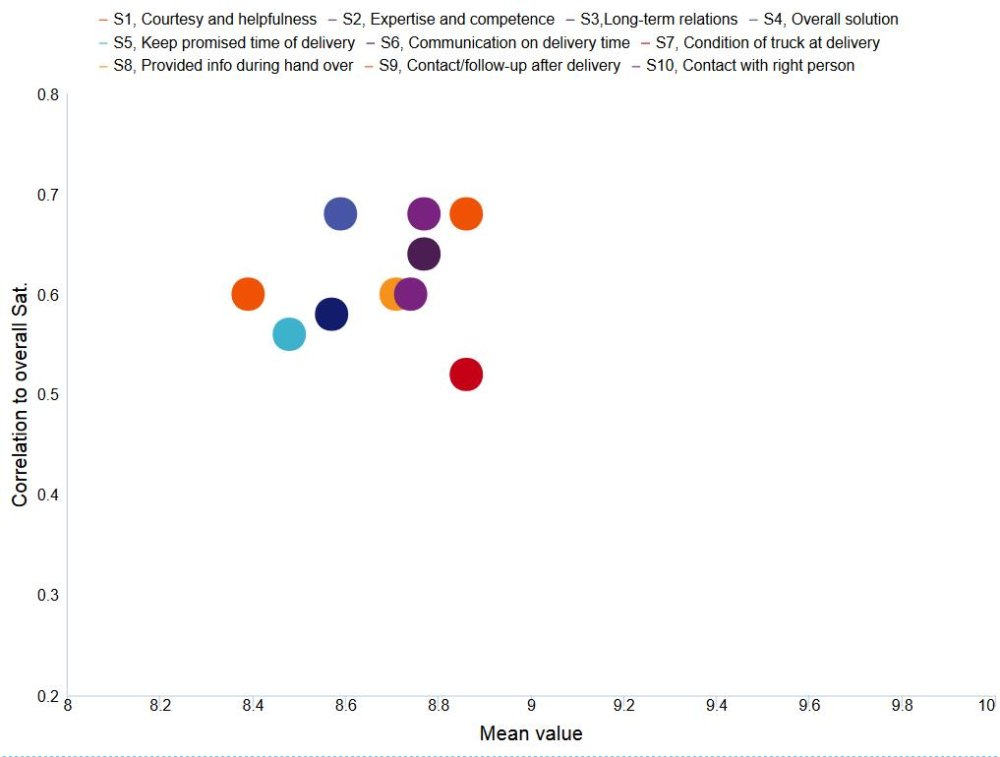Correlation analyses can be performed in the charts, tables and the dynamic icons/shapes in the StoryTeller. The formula used in the calculation is the Pearson formula, and it supports both weighted and unweighted data.

The formula for calculating the Correlation Coefficient “(R)” is shown below. The Coefficient of Determination = (Correlation Coefficient)2
The image below shows the formula for the Correlation Coefficient “(R)”.X = Values from the variable shown in the chart/table
Y = Values from the variable the test is made against
W = The weight of the respondent

When setting up the calculation, you can select if the “Correlation Coefficient” “(R)” or the “Coefficient of Determination” (R2) will be calculated. The output of the tests works in exactly the same way as other calculation types. This means that it can be shown in any chart type or table.

Since the Correlation Analysis is a setting per series, you can create tables and charts which show both the Mean value of categorical variables and the belonging R values (or the R2 values) which supports the use of correlation values in a scatter chart, bubble chart, etc. Below a few examples are shown. (example of R-value in a scatter chart)The example of R-value in a table. The table is sorted by the R-value. Positive values have been colored green and negative values red by using the Cell Formatting functionality.